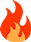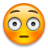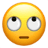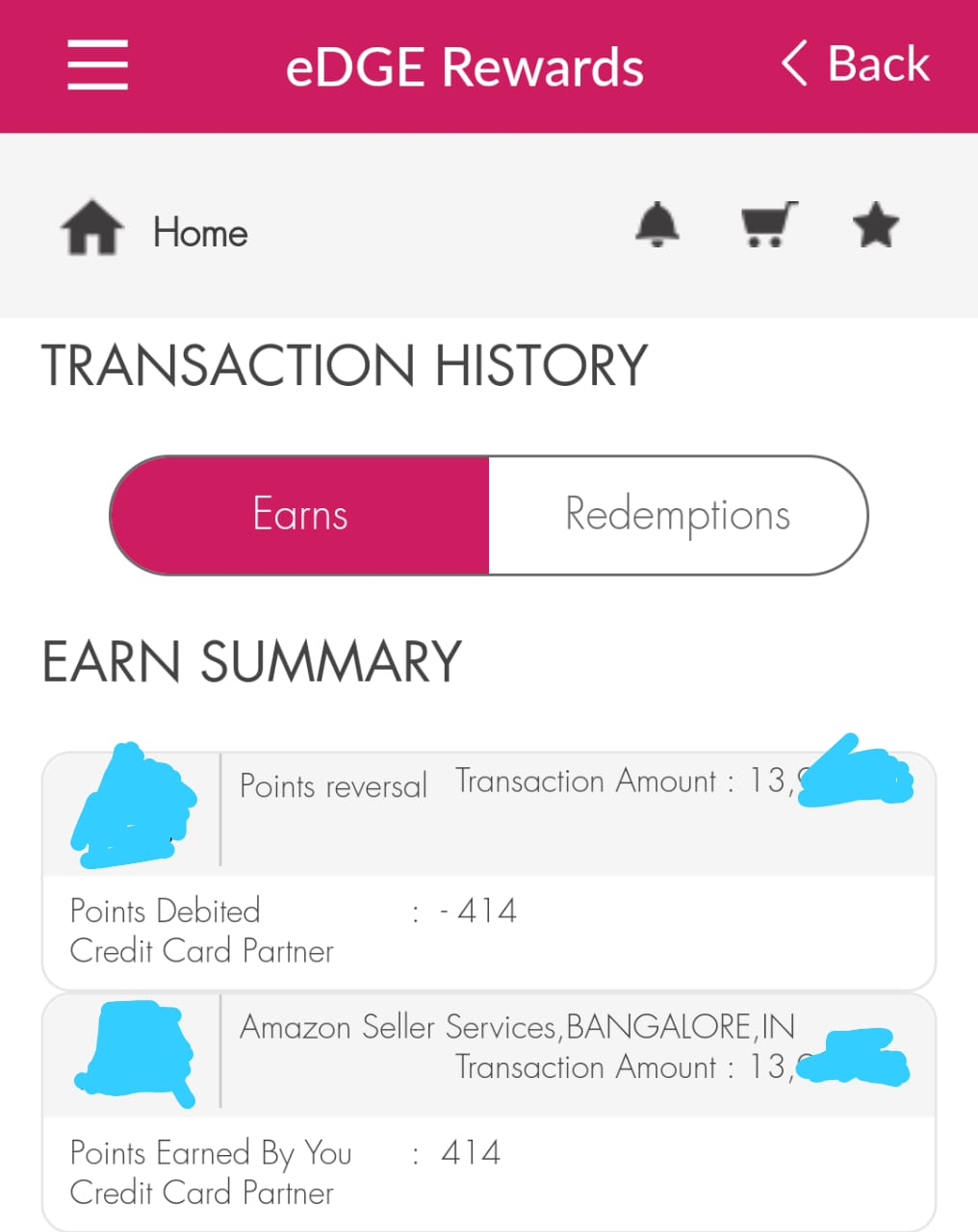# How to calculate GST on no cost EMI??

951°
htzaz

Hi Dimers,
As I’m planning to buy a product (OP7 8+256GB) worth 38000 from Amazon with SBI no cost EMI option. ( 36000 after 2k instant off via SBI CC)
I’m little confused about the actual cost I need to pay to SBI, as they will charge GST on no cost EMI
so could anyone plzz tell me how this GST will me calculated on an EMI of 6000 p.m. for 6 months.
how much would I need to pay for an EMI??

Also please let me know if is it good to take it on no cost EMI or should I go with full payment at once?

13 Dimers
• Sort By
htzaz wrote:

Hi Dimers,
As I’m planning to buy a product (OP7 8+256GB) worth 38000 from Amazon with SBI no cost EMI option. ( 36000 after 2k instant off via SBI CC)
I’m little confused about the actual cost I need to pay to SBI, as they will charge GST on no cost EMI
so could anyone plzz tell me how this GST will me calculated on an EMI of 6000 p.m. for 6 months.
how much would I need to pay for an EMI??

Also please let me know if is it good to take it on no cost EMI or should I go with full payment at once?

the emi cost amzon ll give you as discount .You have to pay the gst to bank .i mean if emi charge is 200+gst amazon ll give the 200 discount.

Expand
Simbha3 wrote:

the emi cost amzon ll give you as discount .You have to pay the gst to bank .i mean if emi charge is 200+gst amazon ll give the 200 discount.

yes bro … I want to know how much gst I need to pay to bank with every EMI

Expand
htzaz wrote:

yes bro … I want to know how much gst I need to pay to bank with every EMI

18% gst on intrest amount ..

Expand
Simbha3 wrote:

18% gst on intrest amount ..

the standard interest rate is 14% from SBI …. could you please explain full tax breakup?

Just check what is the interest amount first. For example, if the interest % is 13% per annum for 6 months EMI on laptop amount Rs. 38000 then interest would be 13% of Rs. 38000 for 1 year = Rs. 4940 but as your EMI is for 6 months it will be Rs. 4940/2 = Rs. 2470 as interest which you might get as upfront discount from Amazon but you will be charged interest from bank.

On top of Rs. 2470 interest over 6 months you will end up paying 18% GST on Rs. 2470 = Rs. 444.6 for 6 months together which is Rs. 74.1 per month.

Hope it’s clear.

raghupro wrote:

Just check what is the interest amount first. For example, if the interest % is 13% per annum for 6 months EMI on laptop amount Rs. 38000 then interest would be 13% of Rs. 38000 for 1 year = Rs. 4940 but as your EMI is for 6 months it will be Rs. 4940/2 = Rs. 2470 as interest which you might get as upfront discount from Amazon but you will be charged interest from bank.

On top of Rs. 2470 interest over 6 months you will end up paying 18% GST on Rs. 2470 = Rs. 444.6 for 6 months together which is Rs. 74.1 per month.

Hope it’s clear.

The GST wouldn’t be 74 every month but 18% of interest of that month so it will be more initially and gradually come down. However total will be 444.6

raghupro wrote:

Just check what is the interest amount first. For example, if the interest % is 13% per annum for 6 months EMI on laptop amount Rs. 38000 then interest would be 13% of Rs. 38000 for 1 year = Rs. 4940 but as your EMI is for 6 months it will be Rs. 4940/2 = Rs. 2470 as interest which you might get as upfront discount from Amazon but you will be charged interest from bank.

On top of Rs. 2470 interest over 6 months you will end up paying 18% GST on Rs. 2470 = Rs. 444.6 for 6 months together which is Rs. 74.1 per month.

Hope it’s clear.

Thanks bro … its pretty clear .
but the interest rate charged by SBI here is 14%
so, 14% interest of 36000 = 5040 p.a
for 6 months (half yearly) it will be 5040/2 = 2520
so GST 18% will be applicable on 2520 = 2520 × 0.18 = 453.6 for 6 months and 75.6 p.m.
so total cost I will be paying as EMI to SBI is 6000+75.6 = 6075.6 pm. for 6 months.
my calculation is correct bro or am I missing something??

Expand

The GST wouldn’t be 74 every month but 18% of interest of that month so it will be more initially and gradually come down. However total will be 444.6

Totally agree, I forgot to incorporate that part.

@htzaz do make a note of this point. Overall across 6 months GST would be Rs. 444.6 but as @adrishya clearly pointed out. For any EMIs in general, the inital payments of EMI would have more being paid for interest and less for principle amount and as time progresses interest component would keep decreasing and principle would keep increasing. Though, overall the EMI amount would be same (principle + interest).

Expand
htzaz wrote:

Thanks bro … its pretty clear .
but the interest rate charged by SBI here is 14%
so, 14% interest of 36000 = 5040 p.a
for 6 months (half yearly) it will be 5040/2 = 2520
so GST 18% will be applicable on 2520 = 2520 × 0.18 = 453.6 for 6 months and 75.6 p.m.
so total cost I will be paying as EMI to SBI is 6000+75.6 = 6075.6 pm. for 6 months.
my calculation is correct bro or am I missing something??

Almost correct.

EMI = 36000/6 = Rs. 6000 + Rs. 2520/6 = Rs. 420 + Rs. 453.6 / 6 = Rs. 75.6
So it would be Rs. 6495.6 per month (do note, as said in previous comment out of the Rs. 6495.6 more would go for interest in initial months, say something like Rs. 900 per month and as months progress it keeps decreasing. Overall you will pay the same Rs. 2520 only as interest though).

You pay the interest to bank each month but Amazon will give it as an upfront discount of Rs. 2520 when you make payment making it effectively no cost EMI. Do check the amount being shown during checkout to cross verify.

so ideally I will be paying approx 500 more in the name of GST on no cost EMI,
either this way or that way, so it would be good to pay at once ??

can i first buy on no cost emi than after 15 days pay the loan amount in full and close the loan?
like if i buy an something at no cost emi and amazon give 30k item at 27.5k after adjusting for 2.5k interest, so can i just pay 27.5k to bank and close the emi?

htzaz wrote:

so ideally I will be paying approx 500 more in the name of GST on no cost EMI,
either this way or that way, so it would be good to pay at once ??

Yes

Brother as per my calculation your total interest amount would be 1327. So u have to pay 18% gst on 1327 that cones to appx 240

Please check how much amount Amazon deducting for 36000 item on no cost 3 month emi.

hiteshu wrote:

Brother as per my calculation your total interest amount would be 1327. So u have to pay 18% gst on 1327 that cones to appx 240

is it total or per month I need to pay 240 extra?

Expand
htzaz wrote:

is it total or per month I need to pay 240 extra?

Total

Expand
hiteshu wrote:

Total

could u please explain ur calculation breakup ?
it would be easy to understand from ur point of view.

But u don’t get reward points on emi transaction

Expand
htzaz wrote:

could u please explain ur calculation breakup ?
it would be easy to understand from ur point of view.

Derive Present Value of 36000 and difference is your interest part. U have to pay gst on it

Use PV formula in excel

hiteshu wrote:

But u don’t get reward points on emi transactionreally ?? r u talking about SBI CC reward points?
Expand
hiteshu wrote:

Derive Present Value of 36000 and difference is your interest part. U have to pay gst on it

Use PV formula in excel

I’m not getting uExpand
htzaz wrote:really ?? r u talking about SBI CC reward points?

No bank gives reward points on EMI AFAIK.

Cc: @a2zdeals

Expand
htzaz wrote:

I’m not getting uTry any product of rs 36000 on amazon and see how much interest they are deducting.
Multiply that interest amount with 18 and devide with 100. It will your gst

Expand
Tejaa wrote:

No bank gives reward points on EMI AFAIK.

Cc: @a2zdeals

htzaz wrote:

so ideally I will be paying approx 500 more in the name of GST on no cost EMI,
either this way or that way, so it would be good to pay at once ??

If you don’t have any crunch right now then go for full payment. No point of availing this no cost emi unless they’re giving you 2k discount only on EMI and not on full payment.

Most of above calculations are wrong except one guy.
Here is breakdown
Principal amount 34576
Interest (upfront discount) 1417
Gst total 255
1st month 72.18
2nd month 60.48
3rd 48.60
4th 36.72
5th 24.66
6th 12.42
Ps there may be error of + 10 or -10 because of decimal places.
To pay least gst buy on 24th of this or any month, since for 1st month emi interest will be for just 10 days.

Go for emi because if you invest 36000 in safest investment(with easy liquidity), you will gain more than 255 rs

Expand
htzaz wrote:

No bank but Yes Bank gives points on EMI transactions@Tejaa
@hiteshu

Few more banks give so that part is wrong

As far as SBI card is concerned:

Will I earn reward points for EMI transactions?

Customers will not receive any reward points for booking a Flexipay EMI. You will earn reward points every time you make a transaction using your SBI Card (excluding fuel & cash transactions).

What would happen to my reward points if I convert a transaction into EMI?

Converting a transaction into an EMI will have no effect on reward points you earn.

Expand
Tejaa wrote:

No bank gives reward points on EMI AFAIK.

Cc: @a2zdeals

YES bank,AXIS bank,HDFC (not sure) will give reward points.. ICICI,kotak they wont give reward points for EMI transactions

Axis bank doesn’t. They reverse points incase of EMI.